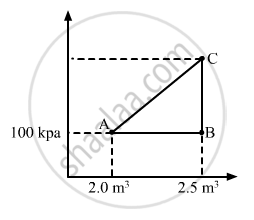Advertisement Remove all ads

# A Gas is Initially at a Pressure of 100 Kpa and Its Volume is 2.0 M3. Its Pressure is Kept Constant and the Volume is Changed from 2.0 M3 to 2.5 M3. - Physics

Sum

A gas is initially at a pressure of 100 kPa and its volume is 2.0 m3. Its pressure is kept constant and the volume is changed from 2.0 m3 to 2.5 m3. Its Volume is now kept constant and the pressure is increased from 100 kPa to 200 kPa. The gas is brought back to its initial state, the pressure varying linearly with its volume. (a) Whether the heat is supplied to or extracted from the gas in the complete cycle? (b) How much heat was supplied or extracted?

Advertisement Remove all ads

#### Solution

(a) Given:-

P1 = 100 kPa,

V1 = 2 m3

V2 = 2.5 m3

∆V = 0.5 m3Work done, W = P∆V

$W = 100 \times {10}^3 \times 0 . 5$

$W = 5 \times {10}^4 J$

WAB = Area under line AB = 5 × 104 J

If volume is kept constant for line BC, then ∆V = 0.

WBC = P∆V = 0

Work done while going from point B to C = 0

When the system comes back to the initial point A from C, work done is equal to area under line AC.

WCA = Area of triangle ABC + Area of rectangle under line AB

Total work done, W = Area enclosed by the ABCA

W = WAC - WAB

From the graph, we see that the area under AC is greater than the area under AB. We also see that heat is extracted from the system as change in the internal energy is zero.

(b) Amount of heat extracted = Area enclosed under ABCA

$= \frac{1}{2} \times 0 . 5 \times 100 \times {10}^3 = 25000 J$

Concept: Heat, Internal Energy and Work
Is there an error in this question or solution?
Advertisement Remove all ads

#### APPEARS IN

HC Verma Class 11, Class 12 Concepts of Physics Vol. 2
Chapter 4 Laws of Thermodynamics
Q 16 | Page 63
Advertisement Remove all ads
Advertisement Remove all ads
Share
Notifications

View all notifications

Forgot password?